# Using Excel's RANK function

Excel contains a number of useful functions for ranking values in a range — in other words, figuring out which value is the highest, the second highest, etc. But there are a number of functions used for ranking, and the difference between them can often be confusing. In this article, we'll explain Excel's RANK function and talk about some of its variants — including RANK.EQ and RANK.AVG . We'll also discuss what to do in the event of a RANK tie.

## Defining the problem

Before we begin, let's look at an example of when the RANK function might be useful.

Take a look at the spreadsheet below, which shows SnackWorld's number of orders by customers this past March. Let's say that a SnackWorld analyst wanted to add a column to the table showing each customer's rank — in other words, who is the best customer, the second-best customer, etc., by number of orders placed. How would we do this?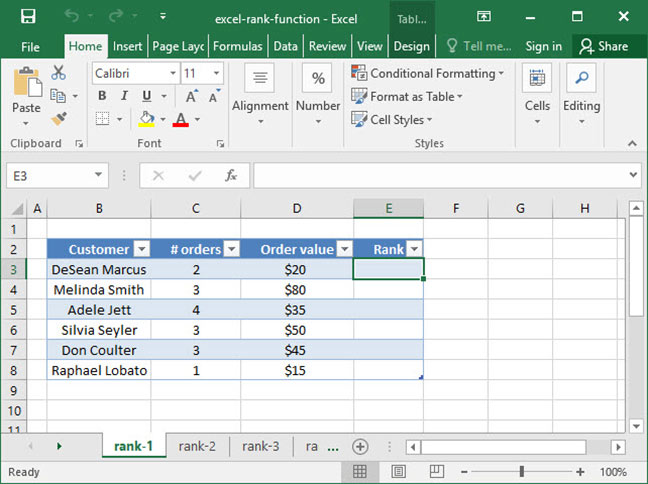## The basic RANK function

We can solve our analyst's problem using the RANK function. The syntax of RANK is as follows:

`=RANK(number, range, order (optional))`

RANK will find the rank of the given number (usually contained within a cell reference) within a given range. If you don't specify an optional order argument, or if you use 0 for the order, RANK will rank the higest value in the given range as 1. But, if you specify 1 as the order, RANK will rank the lowest value in the given range as 1.

Let's take a look at the RANK function used in practice to solve the SnackWorld analyst's problem. Recall that we want to use RANK to find each customer's rank by number of orders placed: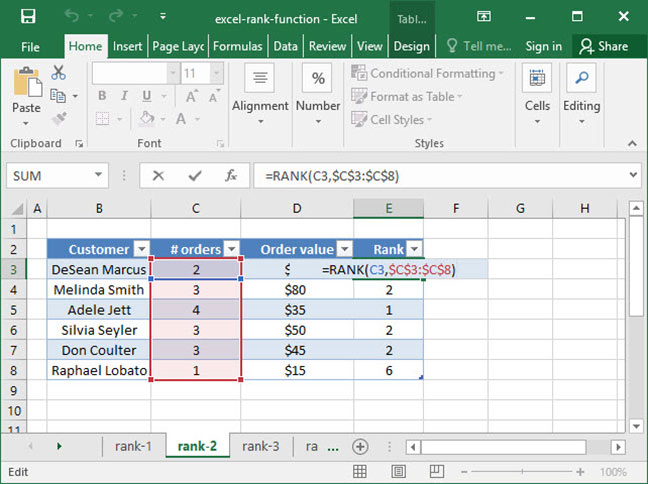`=RANK(C3, \$C\$3:\$C\$8)Output: 5`

For the first cell, the RANK function takes a look at the value in cell C3 and compares it to the values in the # orders column (the range \$C\$3:\$C\$8). Since the value in cell C3, 2 is the fifth highest value in the range \$C\$3:\$C\$8, the formula outputs the number 5.

There are a couple of additional points to note here. First of all, notice that we've used relative and absolute cell references in our formula to lock the range \$C\$3:\$C\$8 and keep rankings consistent throughout the table. If you're unfamiliar with how these references work, check out our tutorial on absolute and relative cell references for more information.

Second, note that the input range to our formula, \$C\$3:\$C\$8, contains three of the same values — 3. In the event of a tie, as we have here, the RANK formula assigns each of these values the same rank — they are all tied for 2nd place. Note that the next highest rank is 5, because there are three values tied for 2nd. Oftentimes, this is an appropriate solution to the tie problem — but in some cases, you may want to break ties. We'll explain how to do this later in the article.

What if we wanted to rank our customers not by number of orders, but by order value? We could easily do that by switching our range to the Order value column: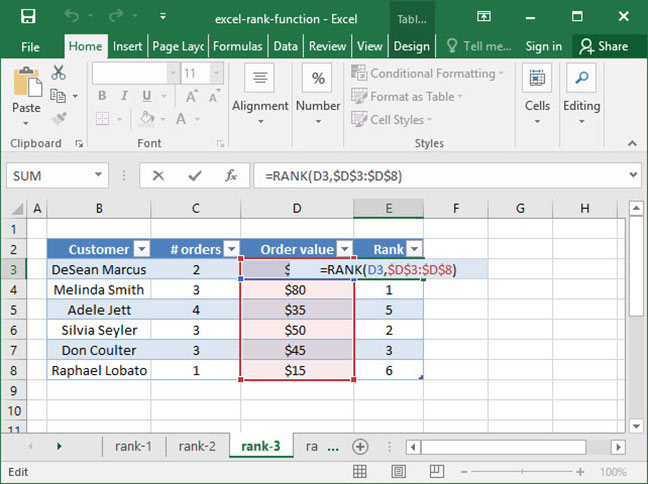`=RANK(D3, \$D\$3:\$D\$8)Output: 4`

The above formula outputs 4, because the order value \$40 is the 4th highest in the range \$D\$3:\$D\$8. Note that we don't have any ties in this case, because all of our Order values are different.

We could also use the optional order parameter to rank our customers from least valuable to most. Let's set order to 1 so that Excel assigns the value 1 to the lowest Order value:

`=RANK(D3, \$D\$3:\$D\$8, 1)Output: 3`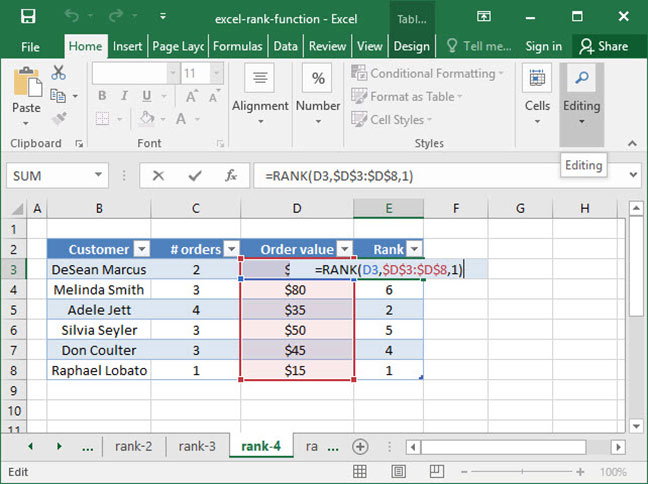The above formula outputs 3, because the order value \$40 is the 3rd lowest in the range \$D\$3:\$D\$8.

## RANK vs. RANK.EQ and RANK.AVG

So you've got a handle on RANK — but what are those RANK.EQ and RANK.AVG functions doing in Excel's inventory? Here's the answer:

RANK and RANK.EQ are exactly the same function, but RANK.EQ was introduced in Excel 2010. This means that if you're writing spreadsheets for versions of Excel prior to 2010, you should always use the RANK function. Otherwise, feel free to use it with RANK.EQ interchangably.

Also introduced in Excel 2010 was the RANK.AVG function. This function is very similar to RANK, except that in the event of a tie in the rankings, instead of setting all of the ranks to the highest tied value, it sets the ranks to the average tied value. Take a look at the following example, in which we've used RANK.AVG to rank our customers by # orders: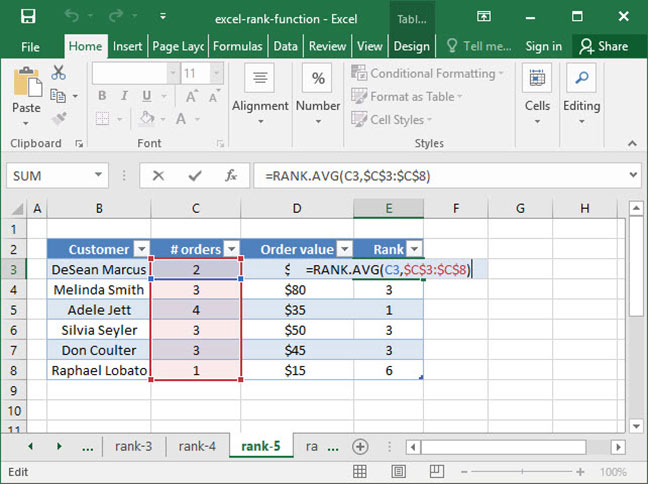`=RANK(C4, \$C\$3:\$C\$8)Output: 3`

Note that since our last example, the output for cell C4 has changed from 2 to 3. This is because we're now using the RANK.AVG function, so Excel takes the average rather than the highest rank in the event of a tie. The cells C4, C6, and C7 are all tied in this rank average at positions 2, 3, and 4. So rather than outputting the highest value, 2, as RANK would do, RANK.AVG outputs 3, the average of 2, 3, and 4.

## Breaking ties with the RANK function

As we've seen, RANK doesn't deal with ties particularly well: it ranks all tie values in the same place. But what if we want to use a "tie breaker" range to determine the winners in the event of a tie?

We can do this, but it requires expanding our spreadsheet a bit and using some tricky number manipulation. Read on to find out how!

Using our SnackWorld example from above, let's say that our analyst wants to rank customers by number of orders placed — but, in the event of a tie in number of orders placed, use the total order value as a tiebreaker. We'll start with the formula we used above to rank customers by number of orders: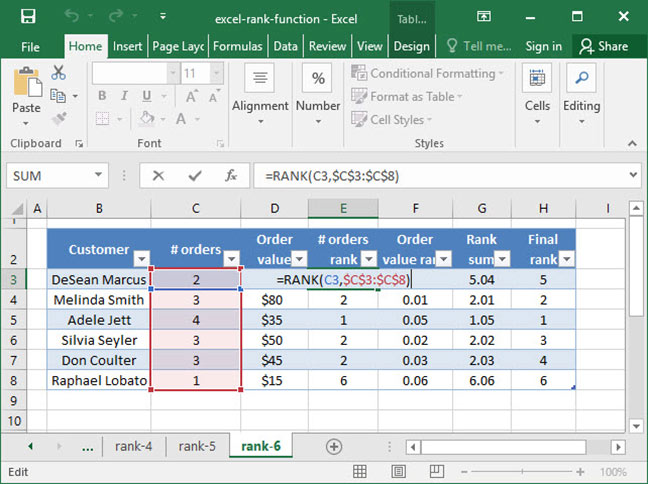`=RANK(C3, \$C\$3:\$C\$8)Output: 5`

Next, we'll add a column to RANK by order value. But there's an extra step: we'll also divide this Order value RANK by 100. We are going to add this decimal to the # orders rank to come up with a combined ranking number that is different for each of our tied customers.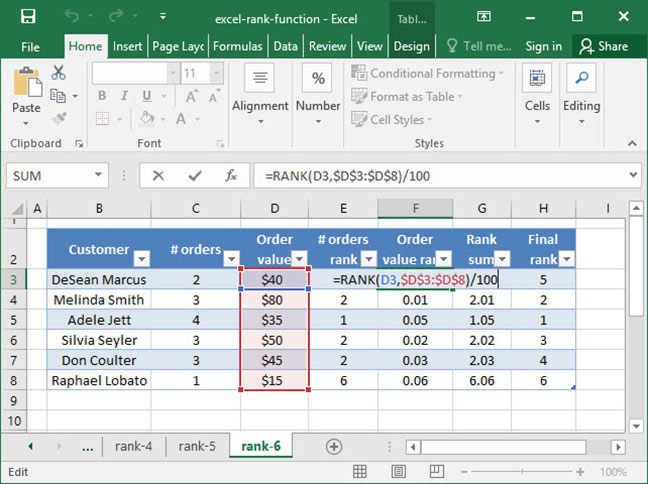`=RANK(D3, \$D\$3:\$D\$8) / 100Output: 0.04`

Next, we create a column that sums the two RANKs: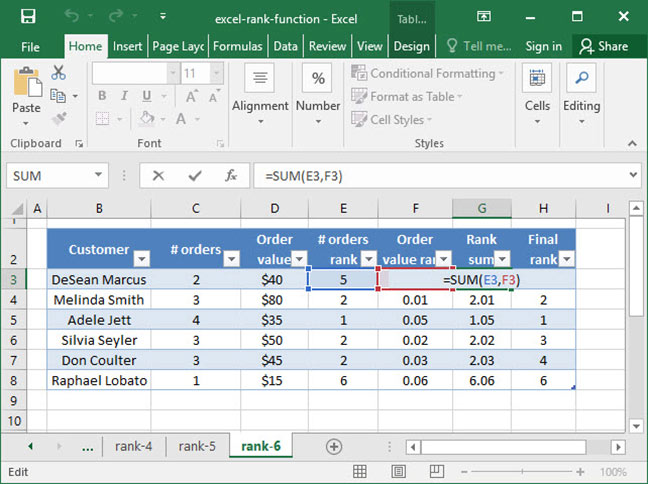`=SUM(E3, F3)Output: 5.04`

Now, we have a list of Rank sums that are all unique. The customers who were initially tied in terms of # orders now have slightly higher or lower numbers due to their different order values.

Finally, we run one last RANK function on this new Rank sum column to generate a final ranked list and break ties. Note that we'll use 1 as the optional order modifier, because we want the lowest Rank sum values to be ranked first: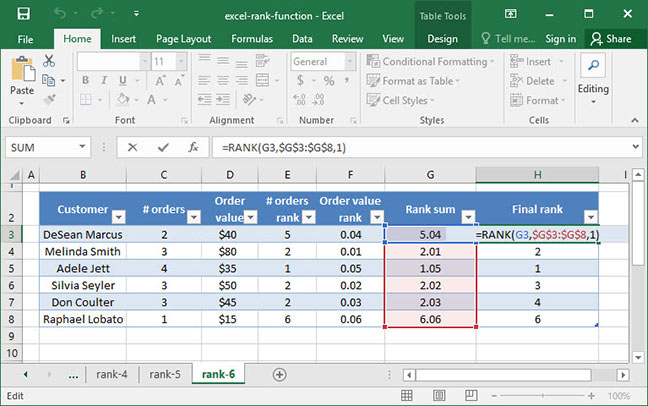`=RANK(G3, \$G\$3:\$G\$8, 1)Output: 5`

And there you have it! As you can see, breaking ties with the RANK function is rather complex, but perfectly possible with a couple of extra columns in your table.

Questions or comments on the RANK function or tie breakers? Let us know in the Comments section below!

## Save an hour of work a day with these 5 advanced Excel tricks

Work smarter, not harder. Sign up for our 5-day mini-course to receive must-learn lessons on getting Excel to do your work for you.

• How to create beautiful table formatting instantly...
• Why to rethink the way you do VLOOKUPs...
• Plus, we'll reveal why you shouldn't use PivotTables and what to use instead...